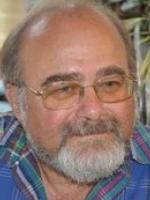# Faculty of Mathematics## Career

• 1999–present: Professor in Numerical Analysis of Differential Equations, Department of Applied Mathematics and Theoretical Physics, University of Cambridge
• 1995–99: Reader in Numerical Analysis of Differential Equations, Department of Applied Mathematics and Theoretical Physics, University of Cambridge
• 1987–95: University Lecturer, Department of Applied Mathematics and Theoretical Physics, University of Cambridge
• 1986–87: Associate professor, Department of Mathematics, University of Arizona
• 1982–86: Senior Research Fellow, King's College, Cambridge
• 1978–82: Junior Research Fellow, King's College, Cambridge

## Research

Arieh Iserles' areas of interest include different aspects of the numerical solution of differential equations and other areas of interest in computational mathematics. In particular, his recent work has focussed on

• Highly oscillatory phenomena and their computation. Here he is interested in particular in effective discretization methods for various types of highly oscillatory integrals in one or more dimensions and in the application of these methods to other problems in numerical analysis, e.g. the solution of rapidly oscillating ordinary differential equations, computation of spectra of Fredholm operators with highly oscillatory kernels and rapid approximation of functions by Birkhoff series.
• Numerical geometric integration, i.e. the discipline concerned with the discretization of differential equations while conserving exactly their invariants. In particular, he is interested in discretization methods for equations that evolve on Lie groups and in homogeneous manifolds. He is also concerned with methods that conserve volume, with symplectic methods and with splitting and composition techniques.
• Effective methods for the approximation of the matrix exponential, in particular approximations that map elements of a Lie algebra to the corresponding Lie group.
• Exponential integrators and asymptotic expansions for ordinary differential equations, with an emphasis of equations that exhibit high oscillation. In particular, he is interested in equations originating in electronic engineering, which possess different interacting scales of nonlinear oscillations.
• Isospectral flows and their features, in particular flows that also possess Lie–Poisson structure. Constructive methods for the computation of the underlying Lie algebra from the structure constants of the Lie–Poisson flow. Issues in matrix analysis that originate in isospectral flows.

## Selected Publications

• A. Iserles & S.P. Nørsett, "Efficient quadrature of highly oscillatory integrals using derivatives", Proc. Royal Soc. A 461 (2005), 1383-1399.
• A.M. Bloch & A. Iserles, "On an isospectral Lie-Poisson system and its Lie algebra", Found. Comp. Maths 6 (2006), 121-144.
• A. Iserles, G.R.W. Quispel & P.S.P. Tse, "B-series methods cannot be volume preserving", BIT 47(2007), 351-378.
• A. Iserles & A. Zanna, "Efficient computation of the matrix exponential by generalized polar decompositions", SIAM J. Num. Anal. 42 (2005), 2218-2256.
• A. Iserles, A First Course in the Numerical Analysis of Differential Equations (2nd edition), Cambridge University Press, 2008. Chinese translation: Springer & Tsing-hua University Press, 2005.
• A. Iserles & S.P. Nørsett, "Quadrature methods for multivariate highly oscillatory integrals using derivatives", Maths Comp 75 (2006), 1233-1258.

## Publications

From high oscillation to rapid approximation V: the equilateral triangle
D Huybrechs, A Iserles, SP Norsett
– IMA Journal of Numerical Analysis
(2011)
31,
755
Simulation technique for RF oscillators
M Condon, A Deano, A Iserles
– Electronics Letters
(2011)
47,
740
From high oscillation to rapid approximation IV: Accelerating convergence
D Huybrechs, A Iserles, SP Norsett
– IMA Journal of Numerical Analysis
(2011)
31,
442
A fast and simple algorithm for the computation of Legendre coefficients.
A Iserles
– Numerische Mathematik
(2011)
117,
529
The computation of the spectra of highly oscillatory Fredholm integral operators
H Brunner, A Iserles, SP Norsett
– Journal of Integral Equations and Applications
(2011)
23,
467
Spectral theory of large Wiener–Hopf operators with complex-symmetric kernels and rational symbols
A Bottcher, S Grudsky, A Iserles
– Mathematical Proceedings of the Cambridge Philosophical Society
(2011)
151,
161
On systems of differential equations with extrinsic oscillation
M Condon, A Deano, A Iserles
– Discrete and Continuous Dynamical Systems
(2010)
28,
1345
On second-order differential equations with highly oscillatory forcing terms
M Condon, A Deano, A Iserles
– Proceedings of the Royal Society A
(2010)
466,
1809
The spectral problem for a class of highly oscillatory Fredholm integral operators
H Brunner, A Iserles, SP Norsett
– IMA Journal of Numerical Analysis
(2010)
30,
108
Asymptotic expansion and quadrature of composite highly oscillatory integrals.
A Iserles, D Levin
– Mathematics of Computation
(2010)
80,
279
• <
• 3 of 17
• >

## Research Groups

Cantab Capital Institute for the Mathematics of Information
Numerical Analysis

F2.06

01223 337891